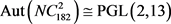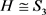﻿ 某类顶点拟本原和二部拟本原的3度对称图的分类

# 某类顶点拟本原和二部拟本原的3度对称图的分类A Classification on a Class of Vertex Quasiprimitive and Bi-Quasiprimitive Cubic Symmetric Graphs

Abstract: Let Γ be a graph and G≤AutΓ. Then Γ is called a G-basic graph, if G is quasiprimitive or bi-quasiprimitive on vertex set V Γ. In this paper, we classify cubic symmetric G-basic graphs of order 2pmqn, where p<q are primes, and m,n≥1.

1. 引言

$X\le \text{Sym}\left(\Omega \right)$ 是一个传递置换群，则称X是拟本原的，如果X的每个极小正规子群都在 $\Omega$ 上传递；称X是二部拟本原的，如果X的每个极小正规子群在 $\Omega$ 上至多有两个轨道并且存在一个极小正规子群作用在 $\Omega$ 上恰有两个轨道。给定一个图 $\Gamma$$G\le \text{Aut}\text{ }\Gamma$，称 $\Gamma$ 是一个G-基图，如果G在顶点集 $V\Gamma$ 上是拟本原的或者二部拟本原的。研究对称图的一般分为以下两步：

2. 预备知识Table 1. 3 degree G-based symmetry diagram of order 2 p m q nTable 2. Point-stabilized subgroups of 3-degree symmetry maps

1) $\Gamma$ 是点传递图，并且 $\text{val}\left(\Gamma \right)=|D|/|H|$

2) $\Gamma$ 是连通图当且仅当 $G=〈D〉$

3) $\Gamma$ 是无向图当且仅当 $D={D}^{-1}$

4) $\Gamma$ 是G-弧传递的当且仅当 $D=H{x}_{i}H\left({x}_{i}\notin H\right)$ 是一个单个的双陪集。

2) 设 $G=\text{PGL}\left(2,13\right)$，则G有一个子群 $H\cong {S}_{3}×{S}_{2}$，且存在一个对合x使得 $|HxH|/|H|=3$$〈H,x〉=G$。于是由引理2.2知陪集图 $\text{Cos}\left(G,H,HxH\right)$ 是一个阶为182的3度对称图，记为 $N{C}_{182}^{2}$，且2) 设 $G=\text{PGL}\left(2,23\right)$，则G有一个子群 $H\cong {S}_{4}$，且存在和一个对合x使得 $|HxH|/|H|=3$$〈H,x〉=G$。于是由引理2.2知陪集图 $\text{Cos}\left(G,H,HxH\right)$ 是一个阶为506的3度对称图，记为 ${C}_{506}$，且 $\text{Aut}\left({C}_{506}\right)\cong \text{PGL}\left(2,23\right)$

2) Smith-Biggs图 $N{C}_{102}$ 是唯一的一个阶为102的3度对称图，且 $\text{Aut}\left(N{C}_{102}\right)\cong \text{PSL}\left(2,17\right)$

3) Coxter-Frucht图 ${C}_{110}$ 是唯一的一个阶为110的3度对称图，且 $\text{Aut}\left({C}_{110}\right)\cong \text{PGL}\left(2,11\right)$

2) 设 $G={S}_{5}$，则G有一个子群，于是通过Magma (  )计算，存在一个阶为20的3度对称图，记为 ${\mathcal{G}}_{20}^{2}$，且 $\text{Aut}\left({\mathcal{G}}_{20}^{2}\right)\cong {S}_{5}×{ℤ}_{2}$

1) 如果 $H\cong {S}_{3}×{S}_{2}$，则存在一个阶为28的3度对称图，记为 ${\mathcal{G}}_{28}^{2}$，且 $\text{Aut}\left({\mathcal{G}}_{28}^{2}\right)\cong \text{PGL}\left(2,7\right)$

2) 如果 $H\cong {S}_{3}$，则存在两个阶为56的3度对称图，分别记为 ${\mathcal{G}}_{56}^{2}$${\mathcal{G}}_{56}^{3}$，且 $\text{Aut}\left({\mathcal{G}}_{56}^{2}\right)\cong \text{PGL}\left(2,7\right)$$\text{Aut}\left({\mathcal{G}}_{56}^{3}\right)\cong \text{PGL}\left(2,7\right)×{ℤ}_{2}$

2) 设 $G=\text{Aut}\left(\text{PSL}\left(2,25\right)\right)$，则G有一个子群 $H\cong {S}_{4}×{S}_{2}$，由Magma (  )可知，存在一个阶为650的3度对称图，记为 ${\mathcal{G}}_{650}^{2}$，且 $\text{Aut}\left({\mathcal{G}}_{650}^{2}\right)\cong \text{Aut}\left(\text{PSL}\left(2,25\right)\right)$

2) 设 $G=\text{Aut}\left(\text{PSL}\left(3,3\right)\right)$，则G有一个子群 $H\cong {S}_{4}×{S}_{2}$，故由Magma (  )计算，存在一个阶为234的3度对称图，记为 ${\mathcal{G}}_{234}^{2}$，且 $\text{Aut}\left({\mathcal{G}}_{234}^{2}\right)\cong \text{Aut}\left(\text{PSL}\left(3,3\right)\right)$

1) N在 $V\Gamma$ 上半正则， $G/N\le \text{Aut}\left({\Gamma }_{N}\right)$${\Gamma }_{N}$ 是G/N-弧传递的，并且 $\Gamma$${\Gamma }_{N}$ 的正规N-覆盖。

2) $\Gamma$$\left(G,s\right)$ -弧传递的当且仅当 ${\Gamma }_{N}$$\left(G/N,s\right)$ -弧传递的，其中 $1\le s\le 5$$s=7$

3) ${G}_{\alpha }\cong {\left(G/N\right)}_{\delta }$，其中 $\alpha \in V\Gamma$$\delta \in V{\Gamma }_{N}$

3. 定理1.1的证明

1) 如果 $|\pi \left(T\right)|=3$，则 $\left(T,|T|\right)$ 满足表3Table 3. Single group T with 3 prime factors

2) 如果 $|\pi \left(T\right)|=4$，则 $\left(T,|T|\right)$ 满足表4Table 4. Single group T with 4 prime factors

$\frac{1}{2}\left(q+1\right)\left(q-1\right)|{2}^{5}\cdot 3\cdot {r}^{m},$

$\frac{q+1}{2}\cdot \frac{q-1}{2}|{2}^{4}\cdot 3\cdot {r}^{m}.$Table 5. Single group T with three prime factors and its corresponding ( p m , q n )Table 6. Single group T with four prime factors and their corresponding ( p m , q n )

(a) ${G}^{+}$${\Delta }_{i}\left(i=1,2\right)$ 上是拟本原的；

(b) ${G}^{+}$ 有两个正规子群 ${M}_{1}$${M}_{2}$ 满足 ${M}_{1}\cong {M}_{2}$ 且它们在 $V\Gamma$ 半正则。进一步，群 ${M}_{1}×{M}_{2}$${\Delta }_{i}$ 上正则。

 Conder, M.D.E. and Dobcsanyi, P. (2002) Trivalent Symmetric Graphs on up to 768 Vertices. Journal of Combinatorial Mathematics and Combinatorial Computing, 40, 41-63.

 Chao, C.Y. (1971) On the Classification of Symmetric Graphs with a Prime Number of Vertices. Transactions of the American Mathematical Society, 158, 247-256.
https://doi.org/10.2307/1995785

 Cheng, Y. and Oxley, J. (1987) On Weakly Symmetric Graphs of Order Twice a Prime. Journal of Combinatorial Theory, Series B, 42, 196-211.
https://doi.org/10.1016/0095-8956(87)90040-2

 Wang, R.J. and Xu, M.Y. (1993) A Classification of Sym-metric Graphs of Order 3p. Journal of Combinatorial Theory, Series B, 58, 197-216.
https://doi.org/10.1006/jctb.1993.1037

 Praeger, C.E., Wang, R.J. and Xu, M.Y. (1993) Symmetric Graphs of Order a Product of Two Distinct Primes. Journal of Combinatorial Theory, Series B, 58, 299-318.
https://doi.org/10.1006/jctb.1993.1046

 Praeger, C.E. and Xu, M.Y. (1993) Vertex-Primitive Graphs of Order a Product of Two Distinct Primes. Journal of Combinatorial Theory, Series B, 59, 245-266.
https://doi.org/10.1006/jctb.1993.1068

 Tutte, W.T. (1947) A Family of Cubical Graphs. Mathematical Pro-ceedings of the Cambridge Philosophical Society, 43, 459-474.
https://doi.org/10.1017/S0305004100023720

 Du, S.F. and Wang, F.R. (2005) Arc-Transitive Cubic Cayley Graphs on PSL(2,p). Science in China, 48, 1927-1308.

 Feng, Y.Q., Kwak, J.H. and Wang, K.S. (2005) Classifying Cubic Symmetric Graphs of Order 8p or 8p2. European Journal of Combinatorics, 26, 1033-1052.
https://doi.org/10.1016/j.ejc.2004.06.015

 Zhou, J.X. and Feng, Y.Q. (2010) Cubic Vertex-Transitive Graphs of Order 2pq. Journal of Graph Theory, 65, 285-302.
https://doi.org/10.1002/jgt.20481

 Biggs, N. (2004) Algebraic Graph Theory. Cambridge University Press, Cambridge.

 Dixon, J.D. and Mortimer, B. (1997) Permu-tation Groups. Spring-Verlag, New York.
https://doi.org/10.1007/978-1-4612-0731-3

 徐明曜. 有限群导引(上)[M]. 北京: 科学出版社, 1999.

 徐明曜. 有限群导引(下)[M]. 北京: 科学出版社, 1999.

 Bosma, W., Cannon, J. and Playoust, C. (1997) The MAGMA Algebra System I: The User Language. Journal of Symbolic Com-putation, 24, 235-265.
https://doi.org/10.1006/jsco.1996.0125

 Li, C.H. and Pan, J.M. (2008) Finite 2-Arc-Transitive Abelian Cayley Graphs. European Journal of Combinatorics, 29, 148-158.
https://doi.org/10.1016/j.ejc.2006.12.001

 Praeger, C.E. (1992) An O’Nan-Scott Theorem for Finite Qua-siprimitive Permutation Groups and an Application to 2-Arc-Transitive Graphs. Journal of the London Mathematical Society, 47, 227-239.
https://doi.org/10.1112/jlms/s2-47.2.227

 Huppert, B. and Lempken, W. (2000) Simple Groups of Order Divisible by at Most Four Primes. Izvestiya Gomel’skogo Gosudarstvennogo Universiteta Im. F. Skoriny, 16, 64-75.

 Giudici, M., Li, C.H. and Praeger, C.E. (2003) Analyzing Finite Locally s-Arc-Transitive Graphs. The Transactions of the American Mathematical Society, 356, 291-317.
https://doi.org/10.1090/S0002-9947-03-03361-0

 Lu, Z.P., Wang, C.Q. and Xu, M.Y. (2004) On Semisym-metric Cubic Graphs of Order 6p2. Science in China Series A, 47, 1-17.

 Li, C.H., Praeger, C.E., Venkatesh, A. and Zhou, S.M. (2014) Finite Locally-Quasiprimitive Graphs. Algebra Colloquium, 21, 627-634.
https://doi.org/10.1142/S1005386714000571

 Conway, J.H., Curtis, R.T., Norton, S.P., Parker, R.A. and Wilson, R.A. (1985) Atlas of Finite Groups. Oxford University Press, London/New York.

Top